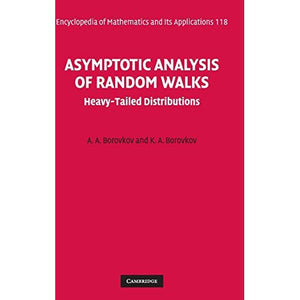># Asymptotic Analysis of Random Walks: Heavy-Tailed Distributions (Encyclopedia of Mathematics and its Applications)

• £59.99
• Save £89

A. A. Borovkov, K. A. Borovkov
Cambridge University Press, 2008-06-12
EAN 9780521881173, ISBN10: 052188117X

Hardcover, 656 pages, 23.4 x 15.6 x 4 cm
Language: English

This book focuses on the asymptotic behaviour of the probabilities of large deviations of the trajectories of random walks with 'heavy-tailed' (in particular, regularly varying, sub- and semiexponential) jump distributions. Large deviation probabilities are of great interest in numerous applied areas, typical examples being ruin probabilities in risk theory, error probabilities in mathematical statistics, and buffer-overflow probabilities in queueing theory. The classical large deviation theory, developed for distributions decaying exponentially fast (or even faster) at infinity, mostly uses analytical methods. If the fast decay condition fails, which is the case in many important applied problems, then direct probabilistic methods usually prove to be efficient. This monograph presents a unified and systematic exposition of the large deviation theory for heavy-tailed random walks. Most of the results presented in the book are appearing in a monograph for the first time. Many of them were obtained by the authors.

'This book is a worthy tribute to the amazing fecundity of the structure of random walks!' Mathematical Reviews